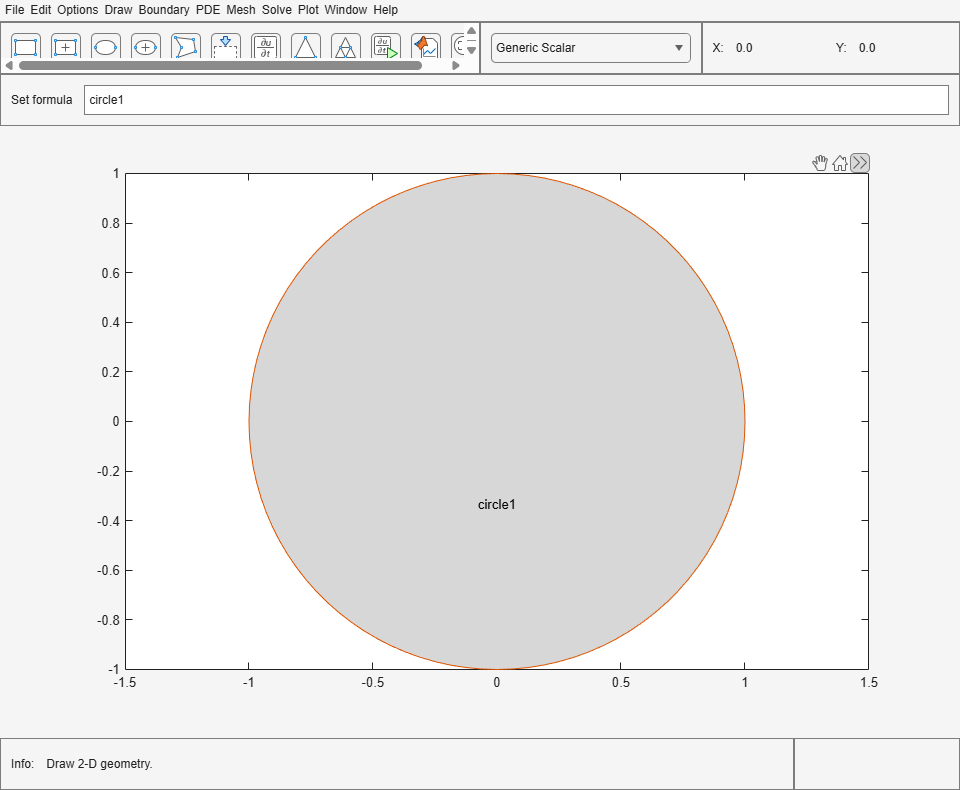pdecirc

Draw circle in PDE Modeler app

Description

example

pdecirc(xc,yc,R) draws a circle with the center at (xc,yc) and the radius R. The pdecirc command opens the PDE Modeler app with the specified circle already drawn in it. If the app is already open, pdecirc adds the specified circle to the app window without deleting any existing shapes.

pdecirc updates the state of the geometry description matrix inside the PDE Modeler app to include the circle. You can export the geometry description matrix from the PDE Modeler app to the MATLAB® Workspace by selecting DrawExport Geometry Description, Set Formula, Labels.... For details on the format of the geometry description matrix, see decsg.

example

pdecirc(xc,yc,R,label) assigns a name to the circle. Otherwise, pdecirc uses a default name, such as C1, C2, and so on.

Examples

collapse all

Open the PDE Modeler app window containing a circle with the center at (0,0) and the radius 1.

pdecirc(0,0,1)

Call the pdecirc command again to draw a circle with the center at (0,0.25) and the radius 0.5. The pdecirc command adds the second circle to the app window without deleting the first.

pdecirc(0,0.25,0.5)Open the PDE Modeler app window containing a circle with the center at (0,0) and the radius 1. Assign the name circle1 to this circle.

pdecirc(0,0,1,'circle1')Input Arguments

collapse all

x-coordinate of the center of the circle, specified as a real number.

Data Types: double

y-coordinate of the center of the circle, specified as a real number.

Data Types: double

Radius of the circle, specified as a positive number.

Data Types: double

Name of the circle, specified as a character vector or string scalar.

Data Types: char | string

Tips

pdecirc opens the PDE Modeler app and draws a circle. If, instead, you want to draw circles in a MATLAB figure window, choose one of these approaches:

• Use the plot command, for example:

t = linspace(0,2*pi);
plot(cos(t),sin(t))
• Use the rectangle function with the Curvature name-value pair set to [1 1].

• Use the Image Processing Toolbox™ viscircles (Image Processing Toolbox) function.